TitleCollege Algebra
Tutorial 12: Complex NumbersLearning Objectives

 After completing this tutorial, you should be able to: Take the principle square root of a negative number. Write a complex number in standard form. Add and subtract complex numbers. Multiply complex numbers. Divide complex numbers.Introduction

 In this tutorial we will be looking at imaginary and complex numbers.  Imaginary numbers allow us to take the square root of negative numbers.  I will take you through adding, subtracting, multiplying and dividing complex numbers as well as finding the principle square root of negative numbers.  I do believe that you are ready to get acquainted with imaginary and complex numbers.Tutorial

 Imaginary Unit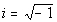where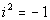This is the definition of an imaginary number. From here on out, anytime that you have the square root of -1 you can simplify it as i and anytime you have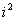you can simplify it as -1.

 Standard Form of Complex Numbers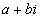Complex numbers are made up of a real number part and an imaginary number part.  In this form, a is the real number part and b is the imaginary number part. Note that either one of these parts can be 0. An example of a complex number written in standard form is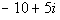.

 Equality of Complex Numbers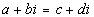if and only if a = c AND b = d.

 In other words, two complex numbers are equal to each other if their real numbers match AND their imaginary numbers match.

 Addition and Subtraction of  Complex Numbers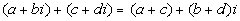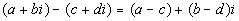In other words, when you add or subtract two complex numbers together, you add or subtract the real number parts together, then add or subtract their imaginary parts together and write it as a complex number in standard form.Example 1: Add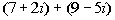.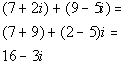*Add the real num. together and the imaginary num. together *Complex num. in stand. formExample 2: Subtract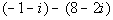.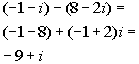*Subtract the real num. together and the imaginary num. together *Complex num. in stand. form

 Multiplying  Complex Numbers

 Step 1:  Multiply the complex numbers in the same manner as polynomials.

 If you need a review on multiplying polynomials, go to Tutorial 6: Polynomials.

 Step 2:  Simplify the expression.

 Add real numbers together and imaginary numbers together. Whenever you have an, use the definition and replace it with -1.

 Step 3:  Write the final answer in standard form.Example 3: Multiply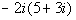.

 Step 1:  Multiply the complex numbers in the same manner as polynomials AND Step 2:  Simplify the expression.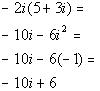*Use dist. prop. to multiply *i squared = -1

 Step 3:  Write the final answer in standard form.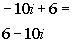*Complex num. in stand. formExample 4: Multiply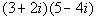.

 Step 1:  Multiply the complex numbers in the same manner as polynomials AND Step 2:  Simplify the expression.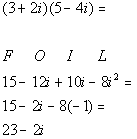*Use FOIL method to multiply *Combine imaginary numbers *i squared = -1

 Step 3:  Write the final answer in standard form.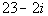*Complex num. in stand. form

 Dividing  Complex Numbers

 Step 1:  Find the conjugate of the denominator.

 You find the conjugate of a binomial by changing the sign that is between the two terms, but keep the same order of the terms.  a + bi and a - bi are conjugates of each other.

 Step 2:  Multiply the numerator and the denominator of the fraction by the conjugate found in Step 1.

 Keep in mind that as long as you multiply the numerator and denominator by the exact same thing, the fractions will be equivalent.  When you multiply complex conjugates together you get: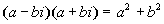Step 3:  Simplify the expression.

 Add real numbers together and imaginary numbers together. Whenever you have an, use the definition and replace it with -1.

 Step 4:  Write the final answer in standard form.Example 5: Divide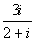.

 Step 1:  Find the conjugate of the denominator.

 In general the conjugate of a + bi is a - bi and vice versa.  So what would the conjugate of our denominator be?  It looks like the conjugate is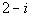.

 Step 2:  Multiply the numerator and the denominator of the fraction by the conjugate found in Step 1 AND Step 3:  Simplify the expression.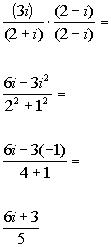*Multiply top and bottom by conj. of den.     **i squared = -1

 Step 4:  Write the final answer in standard form.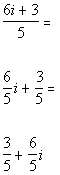*Divide each term of num. by 5     *Complex num. in stand. formExample 6: Divide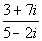.

 Step 1:  Find the conjugate of the denominator.

 In general the conjugate of a + bi is a - bi and vice versa.  So what would the conjugate of our denominator be?  It looks like the conjugate is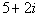.

 Step 2:  Multiply the numerator and the denominator of the fraction by the conjugate found in Step 1 AND Step 3:  Simplify the expression.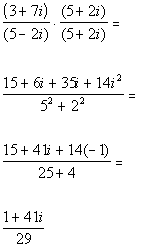*Multiply top and bottom by conj. of den.     **i squared = -1

 Step 4:  Write the final answer in standard form.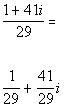*Divide each term of num. by 29 *Complex num. in stand. form

 Principal Square Root  of a Negative Number   For any positive real number b, the principal square root of the negative number, -b, is defined by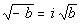Example 7: Simplify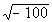.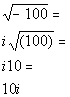*Principal square root of -100 *Complex num. in stand. form (note real num. part is 0)

 Working problems with square roots of  negative numbers.

 In other words use the definition of principal square roots of negative numbers before performing any operations.

 Step 2:  Perform the indicated operation.

 Step 3:  Write the final answer in standard form.Example 8: Perform the indicated operation.  Write answer in standard form.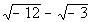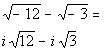*Square root of a negative is i

 Step 2:  Perform the indicated operation AND Step 3:  Write the final answer in standard form.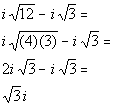*Rewrite 12 as (4)(3) *The square root of 4 is 2 *Subtract like radicals: 2i- i = i *Complex num. in stand. form (note real num. part is 0)Example 9: Perform the indicated operation.  Write answer in standard form.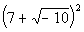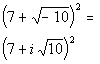*Square root of a negative is i

 Step 2:  Perform the indicated operation AND Step 3:  Write the final answer in standard form.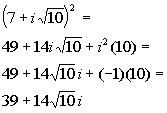*i squared = -1 *Complex num. in stand. formExample 10: Perform the indicated operation.  Write answer in standard form.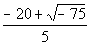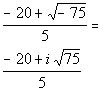*Square root of a negative is i

 Step 2:  Perform the indicated operation AND Step 3:  Write the final answer in standard form.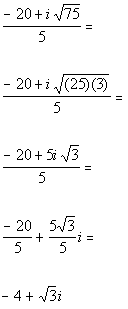*Rewrite 75 as (25)(3)      *The square root of 25 is 5     *Divide each term of num. by 5     *Complex num. in stand. formExample 11: Perform the indicated operation.  Write answer in standard form.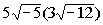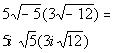*Square root of a negative is i

 Step 2: Perform the indicated operation AND Step 3:  Write the final answer in standard form.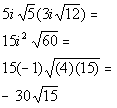*i squared = -1 *Rewrite 60 as (4)(15) *The square root of 4 is 2 *Complex num. in stand. form (note that the imaginary part is 0)Practice Problems

 These are practice problems to help bring you to the next level.  It will allow you to check and see if you have an understanding of these types of problems. Math works just like anything else, if you want to get good at it, then you need to practice it.  Even the best athletes and musicians had help along the way and lots of practice, practice, practice, to get good at their sport or instrument.  In fact there is no such thing as too much practice. To get the most out of these, you should work the problem out on your own and then check your answer by clicking on the link for the answer/discussion for that  problem.  At the link you will find the answer as well as any steps that went into finding that answer.Practice Problems 1a - 1i: Perform the indicated operation. Write the answer in standard form.

 1a.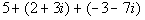(answer/discussion to 1a) 1b.(answer/discussion to 1b) 1c.(answer/discussion to 1c)

 1d.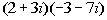(answer/discussion to 1d) 1e.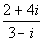(answer/discussion to 1e) 1f.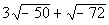(answer/discussion to 1f)

 1g.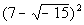(answer/discussion to 1g) 1h.(answer/discussion to 1h) 1i.(answer/discussion to 1i)Need Extra Help on these Topics?

 No appropriate web pages could be found to help you with the topics on this page.   Go to Get Help Outside the Classroom found in Tutorial 1: How to Succeed in a Math Class for some more suggestions.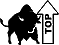Videos at this site were created and produced by Kim Seward and Virginia Williams Trice.
Last revised on Dec. 15, 2009 by Kim Seward.